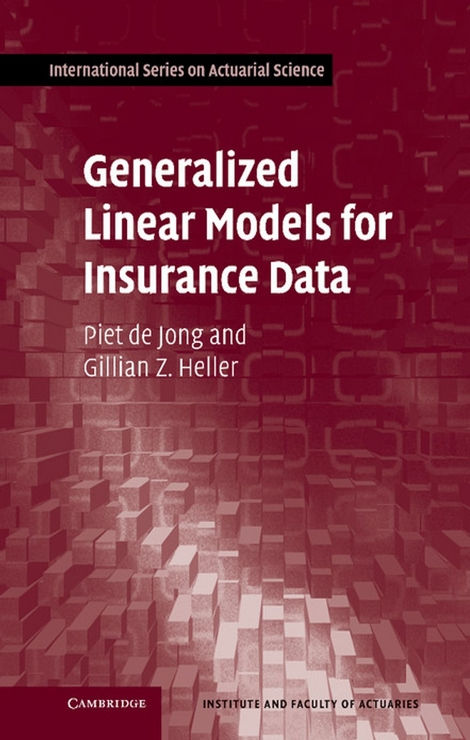# Generalized Linear Models for Insurance DataResumo: Generalized linear modeling is a methodology for modeling relationships between variables. It generalizes the classical normal linear model, by relaxing some of its restrictive assumptions, and provides methods for the analysis of non-normal data. The tools date back to the original article by Nelder and Wedderburn (1972) and have since become part of mainstream statistics, used in many diverse areas of application. This text presents the generalized linear model (GLM) methodology, with applications oriented to data that actuarial analysts are likely to encounter, and the analyses that they are likely required to perform. With the GLM, the variability in one variable is explained by the changes in one or more other variables. The variable being explained is called the “dependent” or “response” variable, while the variables that are doing the explaining are the “explanatory” variables. In some contexts these are called “risk factors” or “drivers of risk.” The model explains the connection between the response and the explanatory variables. Statistical modeling in general and generalized linear modeling in particular is the art or science of designing, fitting and interpreting a model. A statistical model helps in answering the following types of questions: Which explanatory variables are predictive of the response, and what is the appropriate scale for their inclusion in the model? Is the variability in the response well explained by the variability in the explanatory variables? […].

Mais informações aqui.